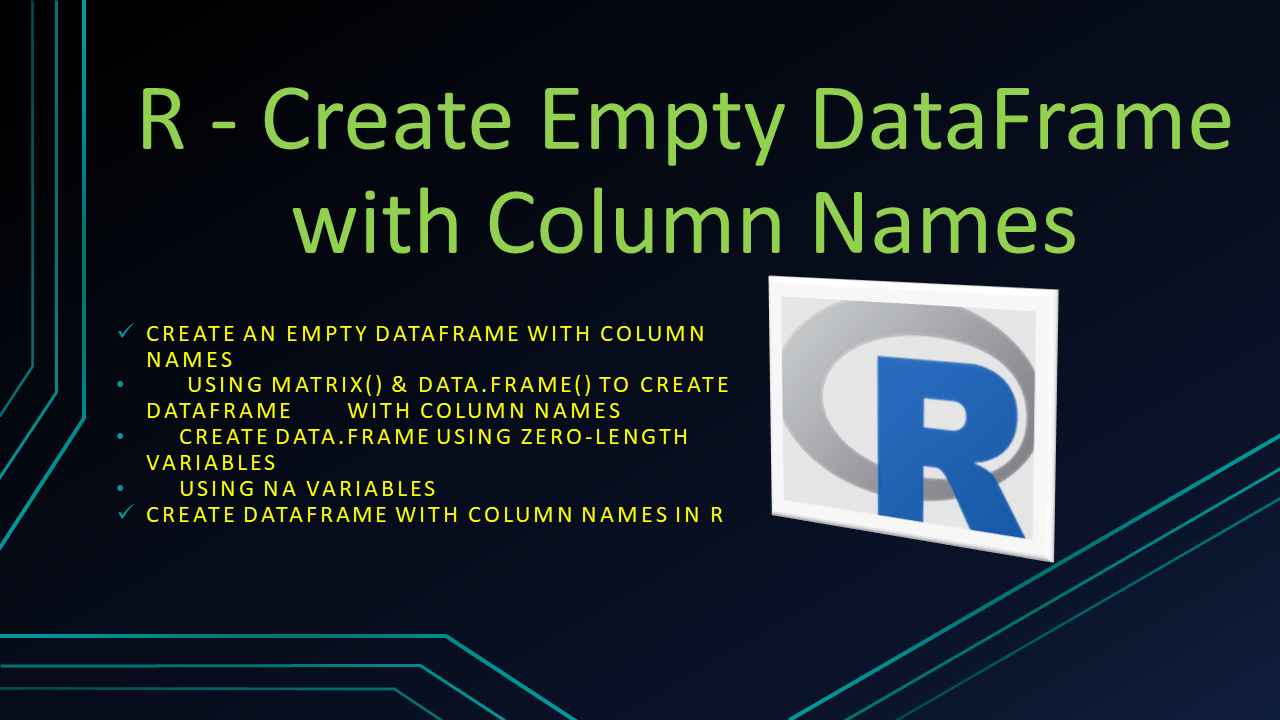# R – Create Empty DataFrame with Column Names?

Let’s see how to create an empty DataFrame with column names in the R programming language by using data.frame() function. Sometimes, you would require to assign a column names while creating a DataFrame or assigning names right after creating it.

Here, I will cover several examples of create an empty DataFrame with column names and create a DataFrame with column names and a few rows.

## 1. Quick Examples of Create DataFrame with Column Names in R

Following are quick examples of how to create an empty DataFrame with column names in R.

``````
# Example 1
#Create a Empty DataFrame with 0 rows and n columns
columns = c("id","name","dob")
df = data.frame(matrix(nrow = 0, ncol = length(columns)))
colnames(df) = columns
df

# Example 2
df2 = data.frame(id=numeric(0),name=character(0),dob=character(0))
df2

# Example 3
df3 = data.frame(id=NA, name=NA, dob=NA)[numeric(0), ]
df3
``````

## 2. Create an Empty DataFrame with Column Names

We are often required to create an empty DataFrame with column names and R provides several ways to achieve this. In this section, I will show different examples of how to create a DataFrame with column names in the R programming language.

### 2.1 Using matrix() & data.frame() to create DataFrame with Column Names

Since we need column names on DataFrame, let’s create a vector with column names first, c() is used to create a Vector in R. Now, let’s create an empty DataFrame by using `data.frame()` function. Finally, print the DataFrame and check if it is really created empty with column names.

``````
# Create a Vector with Columns
columns = c("id","name","dob")

#Create a Empty DataFrame with 0 rows and n columns
df = data.frame(matrix(nrow = 0, ncol = length(columns)))

# Assign column names
colnames(df) = columns

# print DataFrame
print(df)

# Output
# id   name dob
#<0 rows> (or 0-length row.names)

# Display Dimension
dim(df)
# Output
# 0 3
``````

As you see above, our DataFrame has 0 rows with 3 columns `id`, `name` and `dob`.

### 2.2 Create data.frame using Zero-Length Variables

You can also create a DataFrame with column names by using data.frame() and by using zero-length variables.

``````
# Another way
df2 = data.frame(id=numeric(0),name=character(0),dob=character(0))
print(df2)

# Output
# id   name dob
#<0 rows> (or 0-length row.names)
``````

### 2.3 Using NA Variables

Finally, let’s assign a NA to columns in order to create an empty DataFrame with column names.

``````
# Create Empty DataFrame with column names
df3 = data.frame(id=NA, name=NA, dob=NA)[numeric(0), ]
print(df3)

# Output
# id   name dob
#<0 rows> (or 0-length row.names)
``````

## 3. Create DataFrame with Column Names in R

Now let’s see an example of how to create a R DataFrame with column names.

``````
# Create DataFrame
df <- data.frame(
id = c(10,11,12,13),
name = c('sai','ram','deepika','sahithi'),
dob = as.Date(c('1990-10-02','1981-3-24','1987-6-14','1985-8-16'))
)

# Print DataFrame
df
``````

Yields below output.

``````
# Output
id    name        dob
1 10     sai 1990-10-02
2 11     ram 1981-03-24
3 12 deepika 1987-06-14
4 13 sahithi 1985-08-16
``````

## 4. Conclusion

In this article you have learned how to create an empty DataFrame with column names and create an non-empty with column names.

## References

### NNK

SparkByExamples.com is a Big Data and Spark examples community page, all examples are simple and easy to understand and well tested in our development environment Read more ..